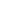## FANDOM

17,784 Pages

 [[File:{{{P1}}}_BW.gif|link={{{P1}}}]][[]][[File:{{{P2}}}_BW.gif|link={{{P2}}}]][[]][[File:{{{P3}}}_BW.gif|link={{{P3}}}]][[]][[File:{{{P4}}}_BW.gif|link={{{P4}}}]][[]][[File:{{{P5}}}_BW.gif|link={{{P5}}}]][[]][[File:{{{P6}}}_BW.gif|link={{{P6}}}]][[]]Lv. - - Lv. - - Lv. ? - Lv. ? - Lv. ? - Lv. ? - Ability: Unknown Ability: Unknown Ability: Unknown Ability: Unknown Ability: Unknown Ability: Unknown Item: ? Item: ? Item: ? Item: ? Item: ? Item: ? - - - - - - - - - - - - - - - - - - - - - - - -

## Template Key

```| game  = (Red/Blue=1, Yellow=2, Gold/Silver=3, Crystal=4, Ruby/Sapphire=5, FireRed/LeafGreen=6, Emerald=7, Diamond/Pearl=8, Platinum=9, HeartGold/SoulSilver=10, Black/White=11)
| NoP   = (# of PKMN 1-6)
|-
| P1    = (1st PKMN)
| GP1   = (1st PKMN Gender [ ♂ / ♀ ] if none leave it blank)
| T1P1  = (1st PKMN TypeA)
| T2P1  = (1st PKMN TypeB)
| LvP1  = (1st PKMN Level)
| AbiP1 = (1st PKMN ability)
| ItmP1 = (1st PKMN item)
| M1P1  = (1st PKMN Move1)
| M2P1  = (1st PKMN Move2)
| M3P1  = (1st PKMN Move3)
| M4P1  = (1st PKMN Move4)
```

## Template

```{{Trainer
| game  =
| NoP   =
|-
| P1    =
| GP1   =
| T1P1  =
| T2P1  =
| LvP1  =
| AbiP1 =
| ItmP1 =
| M1P1  =
| M2P1  =
| M3P1  =
| M4P1  =
|-
| P2    =
| GP2   =
| T1P2  =
| T2P2  =
| LvP2  =
| AbiP2 =
| ItmP2 =
| M1P2  =
| M2P2  =
| M3P2  =
| M4P2  =
|-
| P3    =
| GP3   =
| T1P3  =
| T2P3  =
| LvP3  =
| AbiP3 =
| ItmP3 =
| M1P3  =
| M2P3  =
| M3P3  =
| M4P3  =
|-
| P4    =
| GP4   =
| T1P4  =
| T2P4  =
| LvP4  =
| AbiP4 =
| ItmP4 =
| M1P4  =
| M2P4  =
| M3P4  =
| M4P4  =
|-
| P5    =
| GP5   =
| T1P5  =
| T2P5  =
| LvP5  =
| AbiP5 =
| ItmP5 =
| M1P5  =
| M2P5  =
| M3P5  =
| M4P5  =
|-
| P6    =
| GP6   =
| T1P6  =
| T2P6  =
| LvP6  =
| AbiP6 =
| ItmP6 =
| M1P6  =
| M2P6  =
| M3P6  =
| M4P6  =
}}
```

## Examples

Single Battle: Giovanni

Double Battle: Maxie and Archie

Community content is available under CC-BY-SA unless otherwise noted.